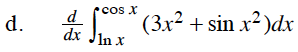### Home > CALC > Chapter 7 > Lesson 7.4.1 > Problem7-178

7-178.
1. Simplify. Homework Help ✎

1.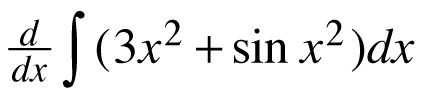2.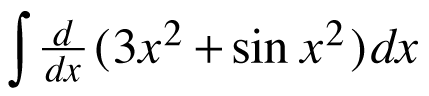3.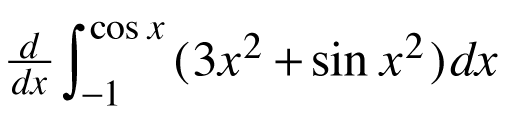4.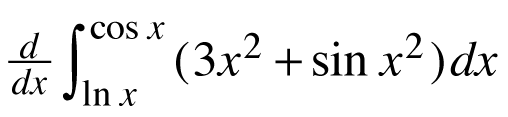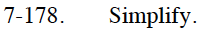All of these problems involve the Fundamental Theorem of Calculus, part 1.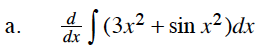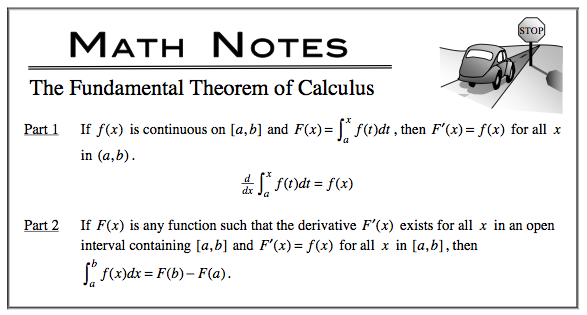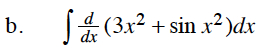Compare and contrast part (a) with part (b). The answers will NOT be identical.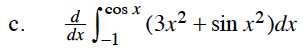The Fundamental Theorem of Calculus, part 1 states that the derivative of an INDEFINITE integral is the same as the original function.
But this is a DEFINITE integral: Remember to multiply by the derivative of the bounds.